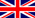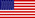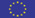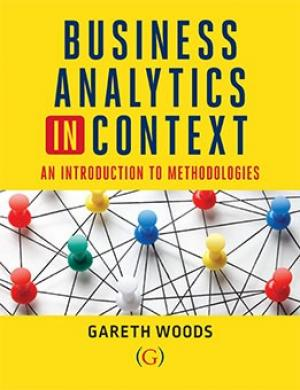Welcome Guest or log-in: emailpassword## An Introduction to Methodologies

#### ISBN: 9781911635147 Paperback; 9781911635154 E-book

Providing you with the mathematical and statistical skills that you will need during your studies, Dr Gareth Woods uses his extensive experience of teaching students requiring additional support to understand key mathematical concepts, to deliver an engaging and accessible text structured around your learning journey from understanding basic concepts, such as algebra, through to applying more advanced techniques including differentiation and optimisation.

Each topic is introduced using a business start-up case study, providing context and showing you how mathematical methods apply to real-life examples. Exercises throughout the book allow you to test your own understanding of the topics covered, and at the end of each chapter you will have the opportunity to apply what you have learnt to a scenario that might be encountered in a professional work environment. The book is supported by online diagnostic tests that allow you to build confidence in using the methodologies contained in the book, prepare for exams and, where needed, to seek further targeted support from your instructors in specific areas to help you progress. Online instructional videos are also provided to further illustrate key concepts and to facilitate self-study.

The content is presented in a work-book style, and space has been provided in the book to allow you to make notes and work through the end of chapter questions. The book then becomes a reference for you as you progress through your studies, and on into professional life. Being able to show your workings to the support staff working in your university’s learning development centre, will help them to address your mathematics support needs quickly and efficiently. Beyond your studies, applicants for graduate positions are often expected to sit psychometric numerical reasoning tests, and having this book as a reference to prepare for and pass these tests would be invaluable in helping you secure that position.

In creating this learning resource, careful consideration has been given to how the online resources integrate with the book to ensure a structured approach to navigating through the content, allowing you to build your confidence in applying the mathematical techniques shown. The learning pathway has been developed so that there are no dead-ends if you find yourself struggling to grasp a particular concept or technique. Mathematics can be a daunting subject for many, and so particular attention has been given to directing you back to content that you may need to engage with again and understand in order to progress further. An illustration of how the this has been achieved can be found in the front of the book.

Publication date: Sept 2020Chapter 1: Numeracy

• 1.1. Order of Operations i.e. BIDMAS/BODMAS
• 1.2. Rounding
• 1.3. Powers and Indices
• 1.4. Scientific Notation
• 1.5. Fractions
• 1.6 Percentages including successive discounts and net present value (NPV)
• 1.7 Ratios
• 1.8 Factors and Multiples

Chapter 2: Algebra

• 2.1. Substitution
• 2.2. Algebraic equations
• 2.3. Inequalities
• 2.4. Expanding brackets
• 2.5. Factorisation
• 2.6. Coordinate geometry
• 2.7. Simultaneous equations, including break even analysis.
• 2.9. Pythagoras theorem
• 2.10. Trigonometry
• 2.11. Logarithms
• 2.12. Sequences and series including geometric series

Chapter 3: Functions

• 3.1. What is a function
• 3.2. Polynomial equations
• 3.3. Exponential functions
• 3.4. Logarithmic function

Chapter 4: Probability

• 4.1. Basic introduction to probability
• 4.2. Condition probability including Bayes Theorem
• 4.3. Probability distributions including Binomial, Poisson and Normal distributions
• 4.4. Decision analysis – decision trees, Maximax, Maximin, Minimax regret, Hurwicz, LaPlace criteria

Chapter 5: Statistics

• 5.1. Representing data – bar charts, line graphs, pie charts and scatter diagrams, histograms
• 5.2. Sampling methods
• 5.3. Measures of location – mean, median, mode.
• 5.4. Measures of location – range, interquartile range, variance, standard deviation.
• 5.5. Correlation
• 5.6. Regression
• 5.7. Multiple regression
• 5.8. Forecasting – time series, moving averages and seasonal effects.
• 5.9. Confidence intervals for means and proportions
• 5.10. Hypothesis testing including t-test, z-test, chi-squared
• 5.11. Type I and II errors

Chapter 6: Calculus

• 6.1. Introduction to differentiation.
• 6.2. Differentiating polynomials, exponentials and logarithms.
• 6.3. Stationary points.
• 6.4. Optimisation problems (using calculus)
• 6.5. Optimisation problems (using linear programming)

Chapter 1: Numeracy

• 1.1. Order of Operations i.e. BIDMAS/BODMAS
• 1.2. Rounding
• 1.3. Powers and Indices
• 1.4. Scientific Notation
• 1.5. Fractions
• 1.6 Percentages including successive discounts and net present value (NPV)
• 1.7 Ratios
• 1.8 Factors and Multiples

Chapter 2: Algebra

• 2.1. Substitution
• 2.2. Algebraic equations
• 2.3. Inequalities
• 2.4. Expanding brackets
• 2.5. Factorisation
• 2.6. Coordinate geometry
• 2.7. Simultaneous equations, including break even analysis.
• 2.9. Pythagoras theorem
• 2.10. Trigonometry
• 2.11. Logarithms
• 2.12. Sequences and series including geometric series

Chapter 3: Functions

• 3.1. What is a function
• 3.2. Polynomial equations
• 3.3. Exponential functions
• 3.4. Logarithmic function

Chapter 4: Probability

• 4.1. Basic introduction to probability
• 4.2. Condition probability including Bayes Theorem
• 4.3. Probability distributions including Binomial, Poisson and Normal distributions
• 4.4. Decision analysis – decision trees, Maximax, Maximin, Minimax regret, Hurwicz, LaPlace criteria

Chapter 5: Statistics

• 5.1. Representing data – bar charts, line graphs, pie charts and scatter diagrams, histograms
• 5.2. Sampling methods
• 5.3. Measures of location – mean, median, mode.
• 5.4. Measures of location – range, interquartile range, variance, standard deviation.
• 5.5. Correlation
• 5.6. Regression
• 5.7. Multiple regression
• 5.8. Forecasting – time series, moving averages and seasonal effects.
• 5.9. Confidence intervals for means and proportions
• 5.10. Hypothesis testing including t-test, z-test, chi-squared
• 5.11. Type I and II errors

Chapter 6: Calculus

• 6.1. Introduction to differentiation.
• 6.2. Differentiating polynomials, exponentials and logarithms.
• 6.3. Stationary points.
• 6.4. Optimisation problems (using calculus)
• 6.5. Optimisation problems (using linear programming)